Next: Frames of reference Up: Newton's laws of motion Previous: Strings, pulleys, and inclines

## Friction

When a body slides over a rough surface a frictional force generally develops which acts to impede the motion. Friction, when viewed at the microscopic level, is actually a very complicated phenomenon. Nevertheless, physicists and engineers have managed to develop a relatively simple empirical law of force which allows the effects of friction to be incorporated into their calculations. This law of force was first proposed by Leonardo da Vinci (1452-1519), and later extended by Charles Augustin de Coulomb (1736-1806) (who is more famous for discovering the law of electrostatic attraction). The frictional force exerted on a body sliding over a rough surface is proportional to the normal reaction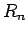at that surface, the constant of proportionality depending on the nature of the surface. In other words,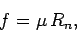(112)

where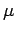is termed the coefficient of (dynamical) friction. For ordinary surfaces,is generally of order unity.

Consider a block of massbeing dragged over a horizontal surface, whose coefficient of friction is, by a horizontal force. See Fig. 32. The weight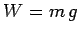of the block acts vertically downwards, giving rise to a reactionacting vertically upwards. The magnitude of the frictional force, which impedes the motion of the block, is simplytimes the normal reaction. Hence,. The acceleration of the block is, therefore,(113)

assuming that. What happens if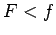: i.e., if the applied forceis less than the frictional force? In this case, common sense suggests that the block simply remains at rest (it certainly does not accelerate backwards!). Hence,is actually the maximum force which friction can generate in order to impede the motion of the block. If the applied forceis less than this maximum value then the applied force is canceled out by an equal and opposite frictional force, and the block remains stationary. Only if the applied force exceeds the maximum frictional force does the block start to move.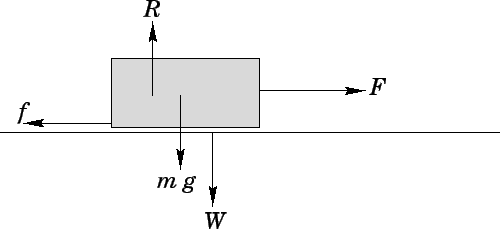Consider a block of masssliding down a rough incline (coefficient of friction) which subtends an angleto the horizontal, as shown in Fig 33. The weight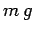of the block can be resolved into components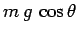, acting normal to the incline, and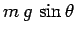, acting parallel to the incline. The reaction of the incline to the weight of the block acts normally outwards from the incline, and is of magnitude. Parallel to the incline, the block is subject to the downward gravitational force, and the upward frictional force(which acts to prevent the block sliding down the incline). In order for the block to move, the magnitude of the former force must exceed the maximum value of the latter, which istime the magnitude of the normal reaction, or. Hence, the condition for the weight of the block to overcome friction, and, thus, to cause the block to slide down the incline, is(114)

or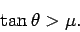(115)

In other words, if the slope of the incline exceeds a certain critical value, which depends on, then the block will start to slide. Incidentally, the above formula suggests a fairly simple way of determining the coefficient of friction for a given object sliding over a particular surface. Simply tilt the surface gradually until the object just starts to move: the coefficient of friction is simply the tangent of the critical tilt angle (measured with respect to the horizontal).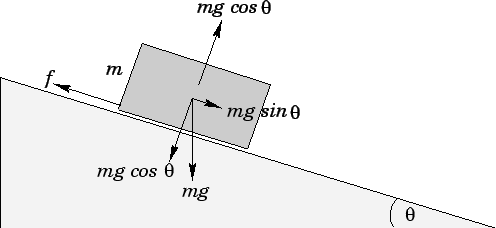Up to now, we have implicitly suggested that the coefficient of friction between an object and a surface is the same whether the object remains stationary or slides over the surface. In fact, this is generally not the case. Usually, the coefficient of friction when the object is stationary is slightly larger than the coefficient when the object is sliding. We call the former coefficient the coefficient of static friction,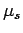, whereas the latter coefficient is usually termed the coefficient of kinetic (or dynamical) friction,. The fact thatsimply implies that objects have a tendency to stick'' to rough surfaces when placed upon them. The force required to unstick a given object, and, thereby, set it in motion, istimes the normal reaction at the surface. Once the object has been set in motion, the frictional force acting to impede this motion falls somewhat totimes the normal reaction.Next: Frames of reference Up: Newton's laws of motion Previous: Strings, pulleys, and inclines
Richard Fitzpatrick 2006-02-02